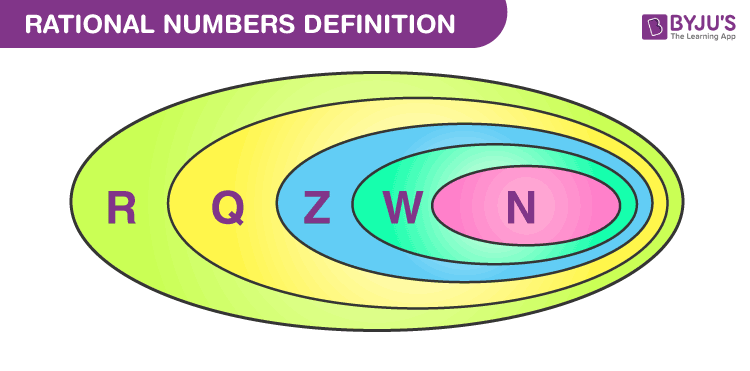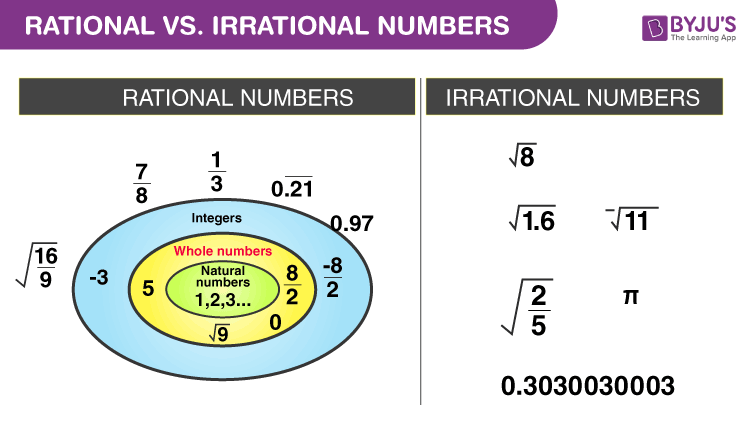# Rational Numbers

In Maths, a rational number is a type of real number, which is in the form of p/q where q is not equal to zero. Any fraction with non-zero denominators is a rational number. Some of the examples of rational numbers are 1/2, 1/5, 3/4, and so on. The number “0” is also a rational number, as we can represent it in many forms such as 0/1, 0/2, 0/3, etc. But, 1/0, 2/0, 3/0, etc. are not rational, since they give us infinite values. Also, check irrational numbers here and compare them with rational numerals.

In this article, we will learn about what is a rational number, the properties of rational numbers along with its types, the difference between rational and irrational numbers, and solved examples. It helps to understand the concepts in a better way. Also, learn the various rational number examples and learn how to find rational numbers in a better way. To represent rational numbers on a number line, we need to simplify and write in the decimal form first.

Let us see what topics we are going to cover here in this article.

## What is a Rational Number?

A rational number, in Mathematics, can be defined as any number which can be represented in the form of p/q where q ≠ 0. Also, we can say that any fraction fits under the category of rational numbers, where the denominator and numerator are integers and the denominator is not equal to zero. When the rational number (i.e., fraction) is divided, the result will be in decimal form, which may be either terminating decimal or the repeating decimal.

### How to identify rational numbers?

To identify if a number is rational or not, check the below conditions.

• It is represented in the form of p/q, where q≠0.
• The ratio p/q can be further simplified and represented in decimal form.

The set of rational numerals:

1. Include positive, negative numbers, and zero
2. Can be expressed as a fraction

Examples of Rational Numbers:

 p q p/q Rational 10 2 10/2 =5 Rational 1 1000 1/1000 = 0.001 Rational 50 10 50/10 = 5 Rational

## Types of Rational Numbers

A number is rational if we can write it as a fraction, where both denominator and numerator are integers and the denominator is a non-zero number.

The below diagram helps us to understand more about the number sets.• Real numbers (R) include all the rational numbers (Q).
• Real numbers include the integers (Z).
• Integers involve natural numbers(N).
• Every whole number is a rational number because every whole number can be expressed as a fraction.

### Standard Form of Rational Numbers

The standard form of a rational number can be defined if it’s no common factors aside from one between the dividend and divisor and therefore the divisor is positive.

For example, 12/36 is a rational number. But it can be simplified as 1/3; common factors between the divisor and dividend is only one. So we can say that rational number ⅓ is in standard form.

### Positive and Negative Rational Numbers

As we know that the rational number is in the form of p/q, where p and q are integers. Also, q should be a non-zero integer. The rational number can be either positive or negative. If the rational number is positive, both p and q are positive integers. If the rational number takes the form -(p/q), then either p or q takes the negative value. It means that

-(p/q) = (-p)/q = p/(-q).

Now, let’s discuss some of the examples of positive and negative rational numbers.

Positive Rational Numbers Negative Rational Numbers
If both the numerator and denominator are of the same sign. If the numerator and denominator are of opposite signs.
All are greater than 0 All are less than 0
Examples of positive rational numbers: 12/17, 9/11 and 3/5 Examples of negative rational numbers: -2/17, 9/-11 and -1/5.

## Arithmetic Operations on Rational Numbers

In Maths, arithmetic operations are the basic operations we perform on integers. Let us discuss here how we can perform these operations on rational numbers, say p/q and s/t.

Addition: When we add p/q and s/t, we need to make the denominator the same. Hence, we get (pt+qs)/qt.

Example: 1/2 + 3/4 = (2+3)/4 = 5/4

Subtraction: Similarly, if we subtract p/q and s/t, then also, we need to make the denominator same, first, and then do the subtraction.

Example: 1/2 – 3/4 = (2-3)/4 = -1/4

Multiplication: In case of multiplication, while multiplying two rational numbers, the numerator and denominators of the rational numbers are multiplied, respectively. If p/q is multiplied by s/t, then we get (p×s)/(q×t).

Example: 1/2 × 3/4 = (1×3)/(2×4) = 3/8

Division: If p/q is divided by s/t, then it is represented as:
(p/q)÷(s/t) = pt/qs

Example: 1/2 ÷ 3/4 = (1×4)/(2×3) = 4/6 = 2/3

## Multiplicative Inverse of Rational Numbers

As the rational number is represented in the form p/q, which is a fraction, then the multiplicative inverse of the rational number is the reciprocal of the given fraction.

For example, 4/7 is a rational number, then the multiplicative inverse of the rational number 4/7 is 7/4, such that (4/7)x(7/4) = 1

## Rational Numbers Properties

Since a rational number is a subset of the real number, the rational number will obey all the properties of the real number system. Some of the important properties of the rational numbers are as follows:

• The results are always a rational number if we multiply, add, or subtract any two rational numbers.
• A rational number remains the same if we divide or multiply both the numerator and denominator with the same factor.
• If we add zero to a rational number then we will get the same number itself.
• Rational numbers are closed under addition, subtraction, and multiplication.

## Rational Numbers and Irrational Numbers

There is a difference between rational and Irrational Numbers. A fraction with non-zero denominators is called a rational number. The number ½ is a rational number because it is read as integer 1 divided by integer 2. All the numbers that are not rational are called irrational. Check the chart below, to differentiate between rational and irrational.Rationals can be either positive, negative or zero. While specifying a negative rational number, the negative sign is either in front or with the numerator of the number, which is the standard mathematical notation. For example, we denote the negative of 5/2 as -5/2.

An irrational number cannot be written as a simple fraction but can be represented with a decimal. It has endless non-repeating digits after the decimal point. Some of the common irrational numbers are:

Pi (π) = 3.142857…

Euler’s Number (e) = 2.7182818284590452…….

√2 = 1.414213…

### How to Find the Rational Numbers between Two Rational Numbers?

There are infinite numbers of rational numbers between two rational numbers. The rational numbers between two rational numbers can be found easily using two different methods. Now, let us have a look at the two different methods.

Method 1:

Find out the equivalent fraction for the given rational numbers and find out the rational numbers in between them. Those numbers should be the required rational numbers.

Method 2:

Find out the mean value for the two given rational numbers. The mean value should be the required rational number. In order to find more rational numbers, repeat the same process with the old and the newly obtained rational numbers.

## Video Lesson on Rational Numbers### Solved Examples

Example 1:

Identify each of the following as irrational or rational: ¾ , 90/12007, 12 and √5.

Solution:

Since a rational number is the one that can be expressed as a ratio. This indicates that it can be expressed as a fraction wherein both denominator and numerator are whole numbers.

• ¾ is a rational number as it can be expressed as a fraction. 3/4 = 0.75
• Fraction 90/12007 is rational.
• 12, also be written as 12/1. Again a rational number.
• Value of  √5 = 2.2360679775…….. It is a non-terminating value and hence cannot be written as a fraction. It is an irrational number.

Example 2:

Identify whether a mixed fraction, 11/2 is a rational number.

Solution:

The Simplest form of 11/2 is 3/2

Numerator = 3, which is an integer

Denominator = 2, is an integer and not equal to zero.

So, yes, 3/2 is a rational number.

Example 3:

Determine whether the given numbers are rational or irrational.

(a) 1.75 (b) 0.01   (c) 0.5  (d) 0.09   (d) √3

Solution:

The given numbers are in decimal format. To find whether the given number is decimal or not, we have to convert it into the fraction form (i.e., p/q)

If the denominator of the fraction is not equal to zero, then the number is rational, or else, it is irrational.

 Decimal Number Fraction Rational Number 1.75 7/4 yes 0.01 1/100 yes 0.5 1/2 yes 0.09 1/11 yes √ 3 ? No

To solve more problems on Rational Numbers register with BYJU’S – The Learning App which provides detailed and step-by-step solutions to all Maths-related concepts. Also, take free tests to score well in your exams.

## Frequently Asked Questions on Rational Numbers

Q1

### What are rational numbers? Give Examples.

A rational number is a number that is in the form of p/q, where p and q are integers, and q is not equal to 0. Some of the examples of rational numbers include 1/3, 2/4, 1/5, 9/3, and so on.

Q2

### What is the difference between rational and irrational numbers?

A rational number is a number that is expressed as the ratio of two integers, where the denominator should not be equal to zero, whereas an irrational number cannot be expressed in the form of fractions. Rational numbers are terminating decimals but irrational numbers are non-terminating and non-recurring. Example of the rational number is 10/2, and for an irrational number is a famous mathematical value Pi(π) which is equal to 3.141592653589…….

Q3

### Is 0 a rational number?

Yes, 0 is a rational number because it is an integer that can be written in any form such as 0/1, 0/2, where b is a non-zero integer. It can be written in the form: p/q = 0/1. Hence, we conclude that 0 is a rational number.

Q4

### Is 7 a rational number?

7 is a rational number because it can be written in the form of a ratio such as 7/1.
Q5

### Is 3.14 a rational number?

Yes, 3.14 is a rational number because it is terminating. But is not a rational number because the exact value of is 3.141592653589793238…which is non terminating non recurring.
Q6

### Find a rational number between 3 and 4.

Rational number between 3 and 4 = (3+4)/2
= 7/2
Q7

### What is the denominator of the rational number?

The denominator of the rational number can be any real number except 0.

Q8

### Is Pi(π) a rational number?

No, Pi (π) is not a rational number. It is an irrational number and its value equals 3.142857…

Test your Knowledge on Rational Numbers

1. You explain things very well and I know because I’m one of the students following and learning the chapters regularly since long

• Please explain me properties of rational numbers

2. So, nice explanation. I khow that because I am download BYJU’s aap in my mobile and I attend all subject class.

3. Really very simple explanation kids can easily get through it thank u I love Byju’s app it makes education fun kids are getting interested in education bcs of Byju’s

Thank you very much

4. so much benificial websit i read only one topic and i am so impressed To much explained with easy words.

5. Very nice illustration
BENEFICAL WEBSITE for study as well as study materisls [book pdf]

6. Fantastic article. Keep up the good work!